# 总结字符串比较函数

最近一段时间一直在重看CLR via C# , 这次把字符串比较的函数总结下。

1.Compare和CompareTo大PK

首先是我们最常用的String.Compare和CompareTo实例方法，先来看看这两个方法：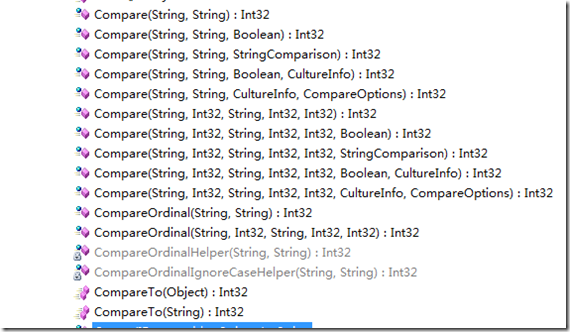我们通过这个可以直观地看到，String的静态方法要比CompareTo多出好多的方法重载，其实这也是两者的最大区别，也就是说String.Compare有着更多的功能选项供我们控制。

其中主要包含着三个方面：

A. 文化信息

B. CompareOptions

C. 比较的开始和结束位置

对于文化信息，我们可以看下Compare的反编译结果：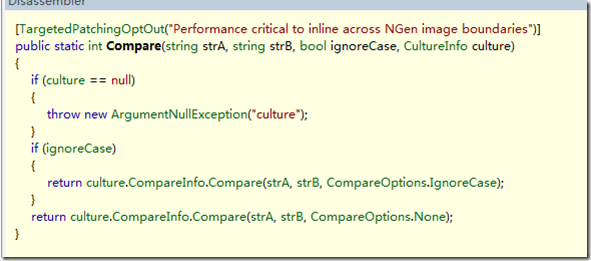对于Compare来说，他会通过传递进来的文化信息来调用对应的比较。

而CompareTo则是：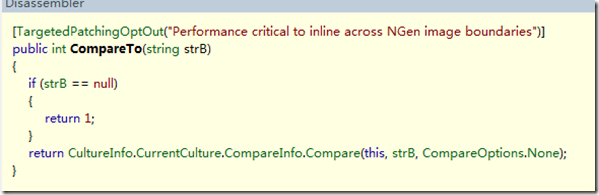CompareTo则会调用与当前线程相关联的文化信息。

对于文化信息来说，还有着这样一个枚举选项：StringComparison:

下面让我们来看下StringComparison枚举：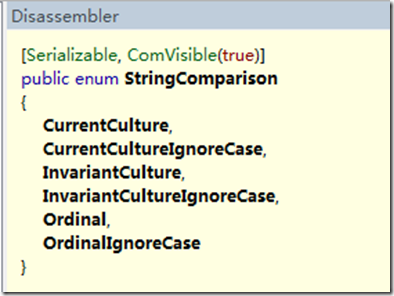对于该枚举，共有如上六个枚举值。该枚举主要对应着当前的文化信息，大小写，以及排序规则。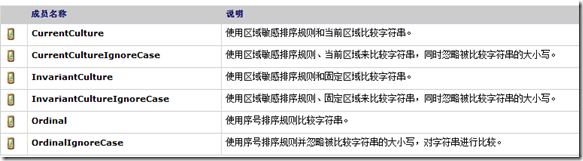这就意味着，如果我们进行国际化的时候，字符串比较必须使用String.Compare静态方法。

下面来看下CompareOptions: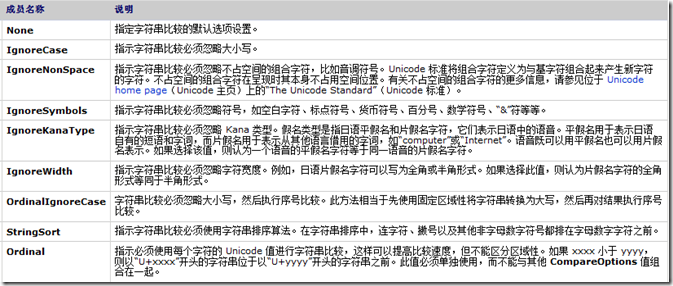最后看下含开始和结束位置的String.Compare方法：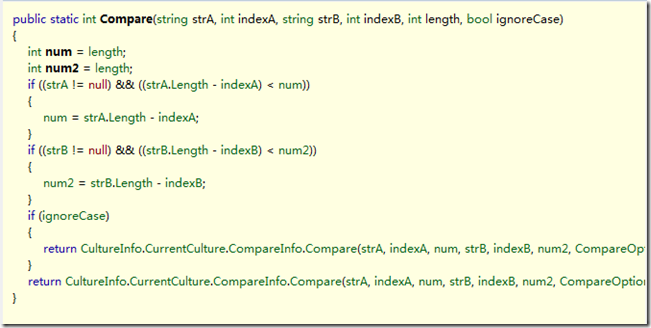方法本身很简单，而方法链的最末端使用的是：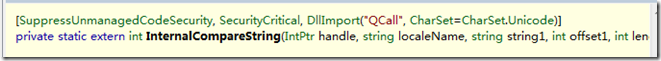一个内部比较字符串的非托管方法，而方法的具体内容，我无从而知，但是可以明确的是，这一定是一个高效的比较算法。

因此，当我们每次SubString的时候，当我们ToLower的时候，我们不妨都在这里使用String.Compare，是不是为我们节省了很多空间，提高了很大效率呢？

因此，我在这里建议，如果可能，我们尽可能地使用String.Compare方法来代替CompareTo方法!

2. 被遗忘的CompareOrdinal

让我们先来看下CompareOrdinal的源码：

```private static unsafe int CompareOrdinalHelper(string strA, string strB)
{
int num = Math.Min(strA.Length, strB.Length);
int num2 = -1;
fixed (char* chRef = &strA.m_firstChar)
{
fixed (char* chRef2 = &strB.m_firstChar)
{
char* chPtr = chRef;
char* chPtr2 = chRef2;
while (num >= 10)
{
if (*(((int*)chPtr)) != *(((int*)chPtr2)))
{
num2 = 0;
break;
}
if (*(((int*)(chPtr + 2))) != *(((int*)(chPtr2 + 2))))
{
num2 = 2;
break;
}
if (*(((int*)(chPtr + 4))) != *(((int*)(chPtr2 + 4))))
{
num2 = 4;
break;
}
if (*(((int*)(chPtr + 6))) != *(((int*)(chPtr2 + 6))))
{
num2 = 6;
break;
}
if (*(((int*)(chPtr + 8))) != *(((int*)(chPtr2 + 8))))
{
num2 = 8;
break;
}
chPtr += 10;
chPtr2 += 10;
num -= 10;
}
if (num2 == -1)
{
goto Label_00F1;
}
chPtr += num2;
chPtr2 += num2;
int num3 = chPtr - chPtr2;
if (num3 != 0)
{
return num3;
}
return (chPtr - chPtr2);
Label_00D7:
if (*(((int*)chPtr)) != *(((int*)chPtr2)))
{
goto Label_00F5;
}
chPtr += 2;
chPtr2 += 2;
num -= 2;
Label_00F1:
if (num > 0)
{
goto Label_00D7;
}
Label_00F5:
if (num > 0)
{
int num4 = chPtr - chPtr2;
if (num4 != 0)
{
return num4;
}
return (chPtr - chPtr2);
}
return (strA.Length - strB.Length);
}
}
}```

方法很长，但是很简单，即使是Reflector 出来的变量名很BT，但是我们也可以大致看个究竟。

他是将整个字符串每5个字符（10个字节）分成一组，然后逐个比较，找到第一个不相同的ASCII码后退出循环。并且求出两者的ASCII码的差。不过我很费解的是微软为什么要把这个实现的如此麻烦。只能等到周一再求解了。

但是在CLR via C#上有这样的话：这个方法比其他方法都要快。我想应该是有一定道理的吧。

所以当我们比较大小的时候，尽量使用CompareOrdinal方法。

3. 常用的Equals方法

先来看Equals实例方法：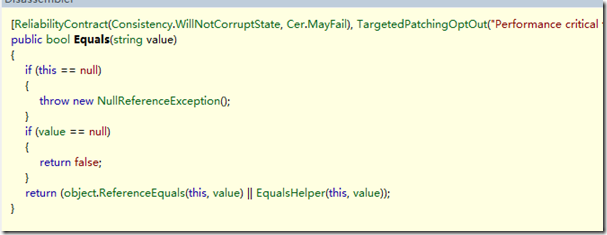方法会首先进行合法性判断，然后比较两者是否指向同一块引用，接下来调用EqualsHelper方法（不清楚微软为什么很沉迷于XXXHelper这个命名，难道XXXHelper这个名词不应该是一个类名么？）

```private static unsafe bool EqualsHelper(string strA, string strB)
{
int length = strA.Length;
if (length != strB.Length)
{
return false;
}
fixed (char* chRef = &strA.m_firstChar)
{
fixed (char* chRef2 = &strB.m_firstChar)
{
char* chPtr = chRef;
char* chPtr2 = chRef2;
while (length >= 10)
{
if ((((*(((int*)chPtr)) != *(((int*)chPtr2))) || (*(((int*)(chPtr + 2))) != *(((int*)(chPtr2 + 2))))) || ((*(((int*)(chPtr + 4))) != *(((int*)(chPtr2 + 4)))) || (*(((int*)(chPtr + 6))) != *(((int*)(chPtr2 + 6)))))) || (*(((int*)(chPtr + 8))) != *(((int*)(chPtr2 + 8)))))
{
break;
}
chPtr += 10;
chPtr2 += 10;
length -= 10;
}
while (length > 0)
{
if (*(((int*)chPtr)) != *(((int*)chPtr2)))
{
break;
}
chPtr += 2;
chPtr2 += 2;
length -= 2;
}
return (length <= 0);
}
}
}```

迷糊了，又是这样的算法，我实在不了解10字节究竟有什么奥秘，周一如果问到答案会对其进行解释。

然而，值得肯定的是，由于是非安全代码的比较，所以效率要比我们用安全代码高得多。

接下来看看Equals静态方法：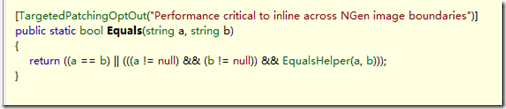(关于==的运算符重载之前有误，下文会解释清楚)

4. 总结

本文主要介绍了String类型的比较方法，也留下了一些疑问，也希望可以得到各位的解答。

0
0